### Trigonometry

Let's explore the sine a little more and see what the graph looks like. To help us get there, let's place the right triangle in a unit circle (a circle with a radius of 1).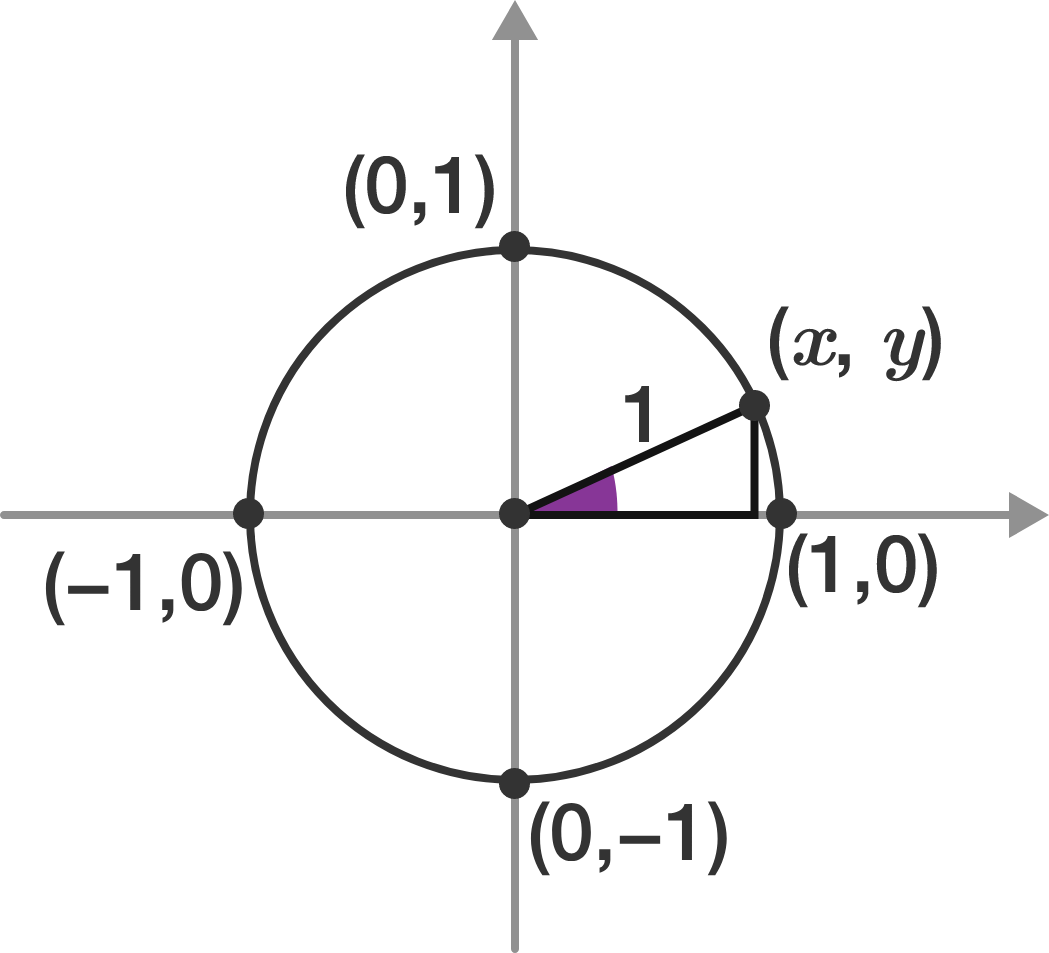This means the hypotenuse will always be 1. If the point on the circle the hypotenuse touches is $(x,y),$ what is the sine of the marked angle?

Hint: Which two sides of the triangle do we use to define the sine of the angle?

# Graphing the Sine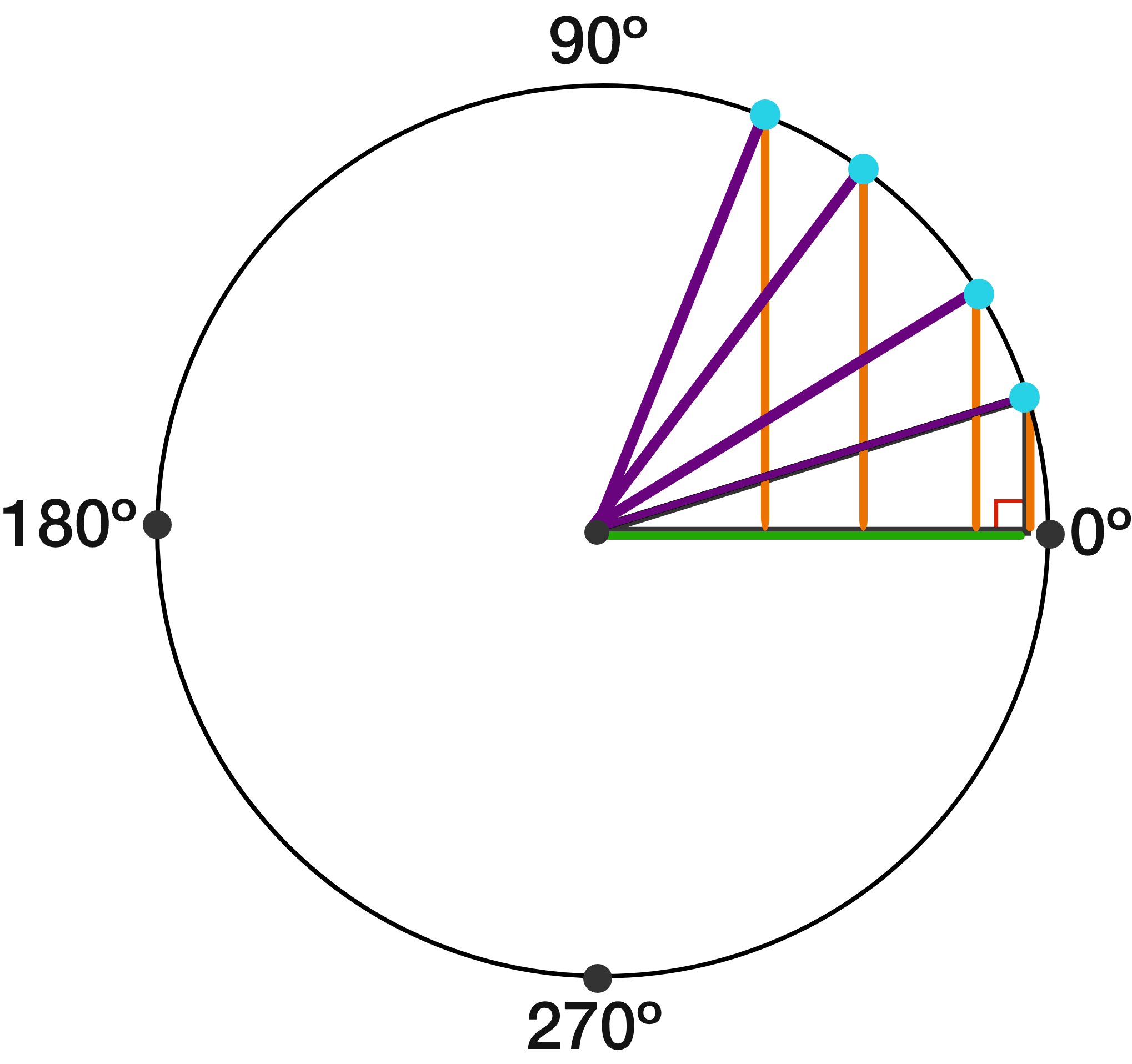Based on the previous question, we can think of taking the sine as the equivalent of keeping track of the $y$-coordinate of a point that rotates around a unit circle.

What does the graph of $f(x)=\sin(x)$ look like?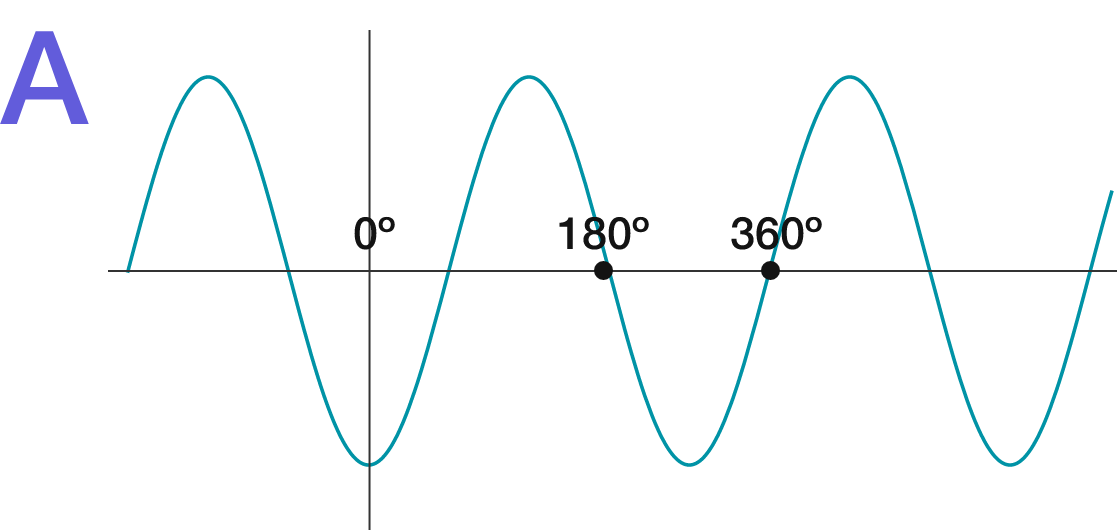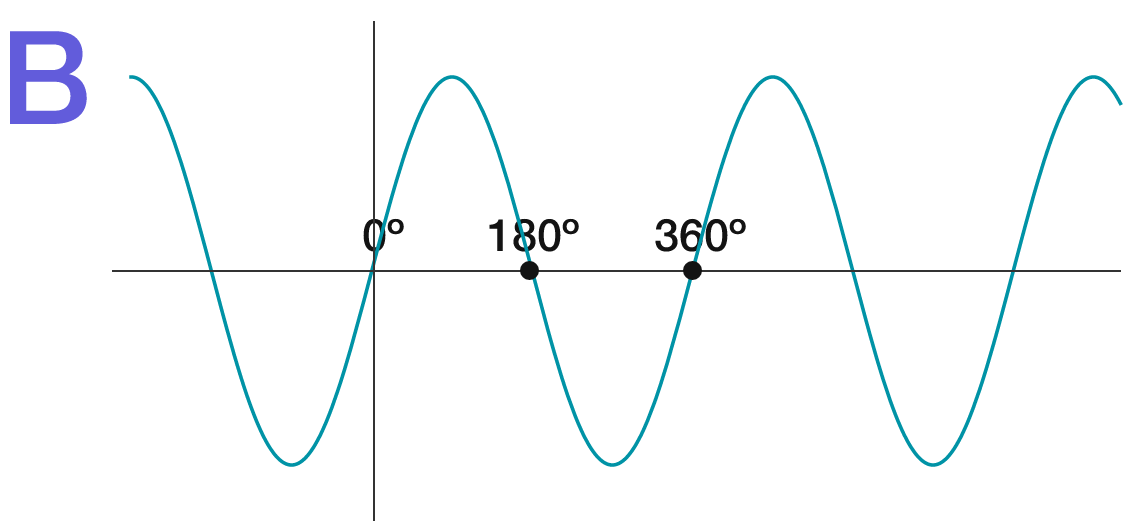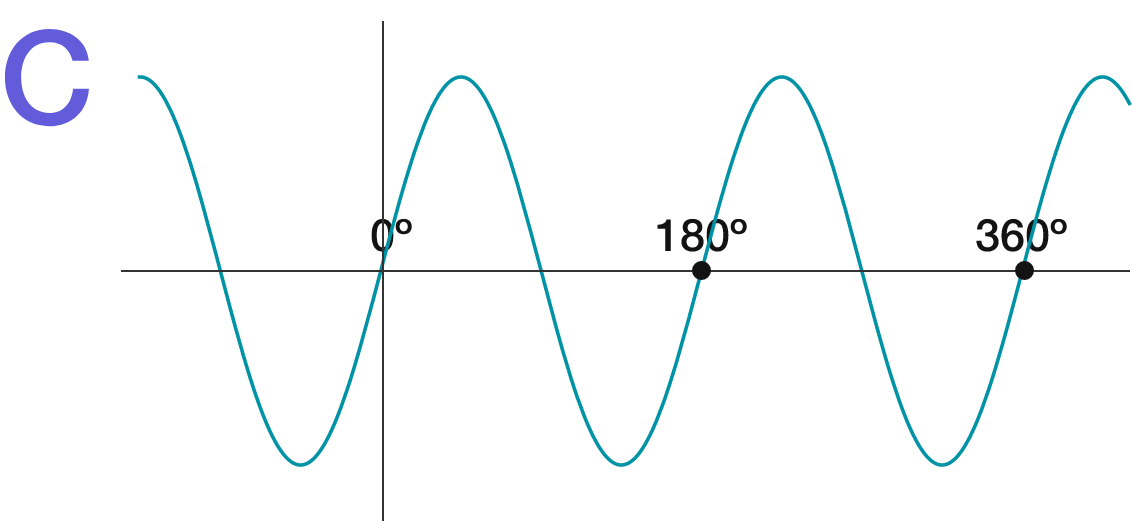# Graphing the Sine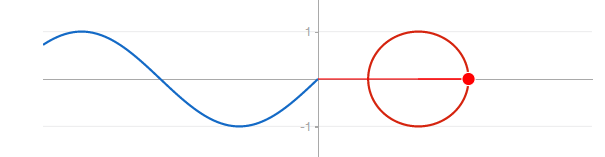Since the sine graph is made by rotating around a unit circle, it cycles every time the full circle is complete.

Which of the answer choices makes a graph identical to that of $f(x)=\sin(x) ?$

Hint: The transformation $f(x - h)$ shifts the graph right by $h .$

# Graphing the Sine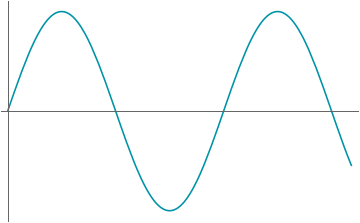By turning the graph of the sine into a wave, we have a representation that matches how radio waves, sound waves, light waves, and many more types of waves act.

Pitch is a way to position notes in music that is "lower" or "higher"; the graph above shows a sound wave that starts low in pitch and gradually increases in pitch.

The graph below shows two sound waves. What is true?

(Note: You can hear these waves in the answer!)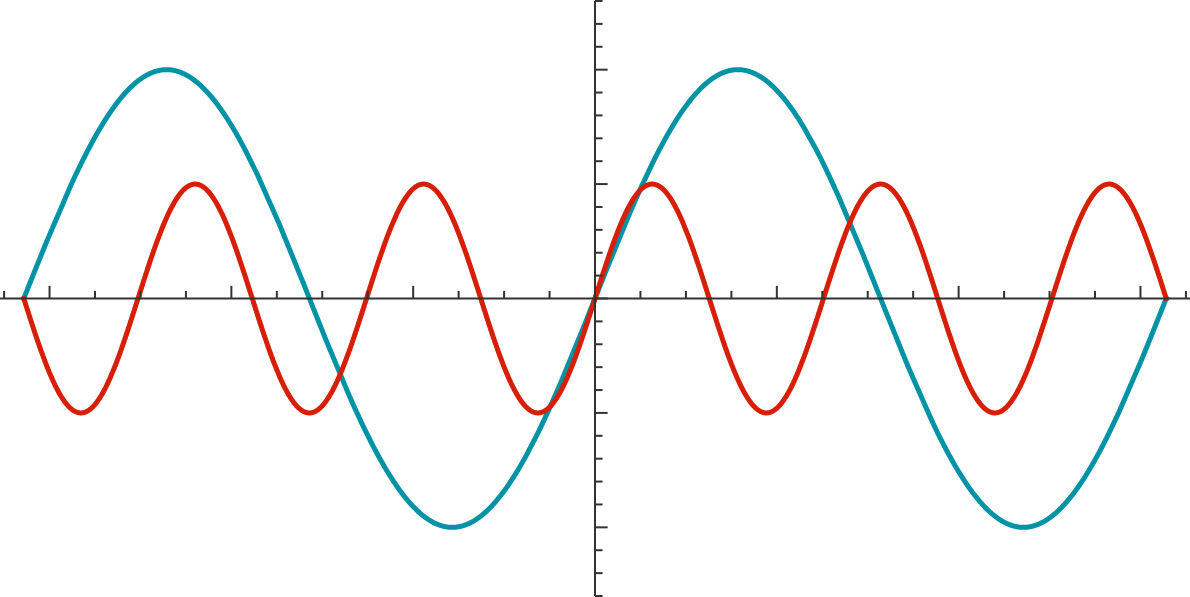# Graphing the Sine

Waves can be combined to form increasingly complex sounds; even the most complex, multi-instrument music amounts to just a single sound wave that comes from adding multiple waves. In other words, no matter how complex, a sum of sine waves will be able to sound like literally anything.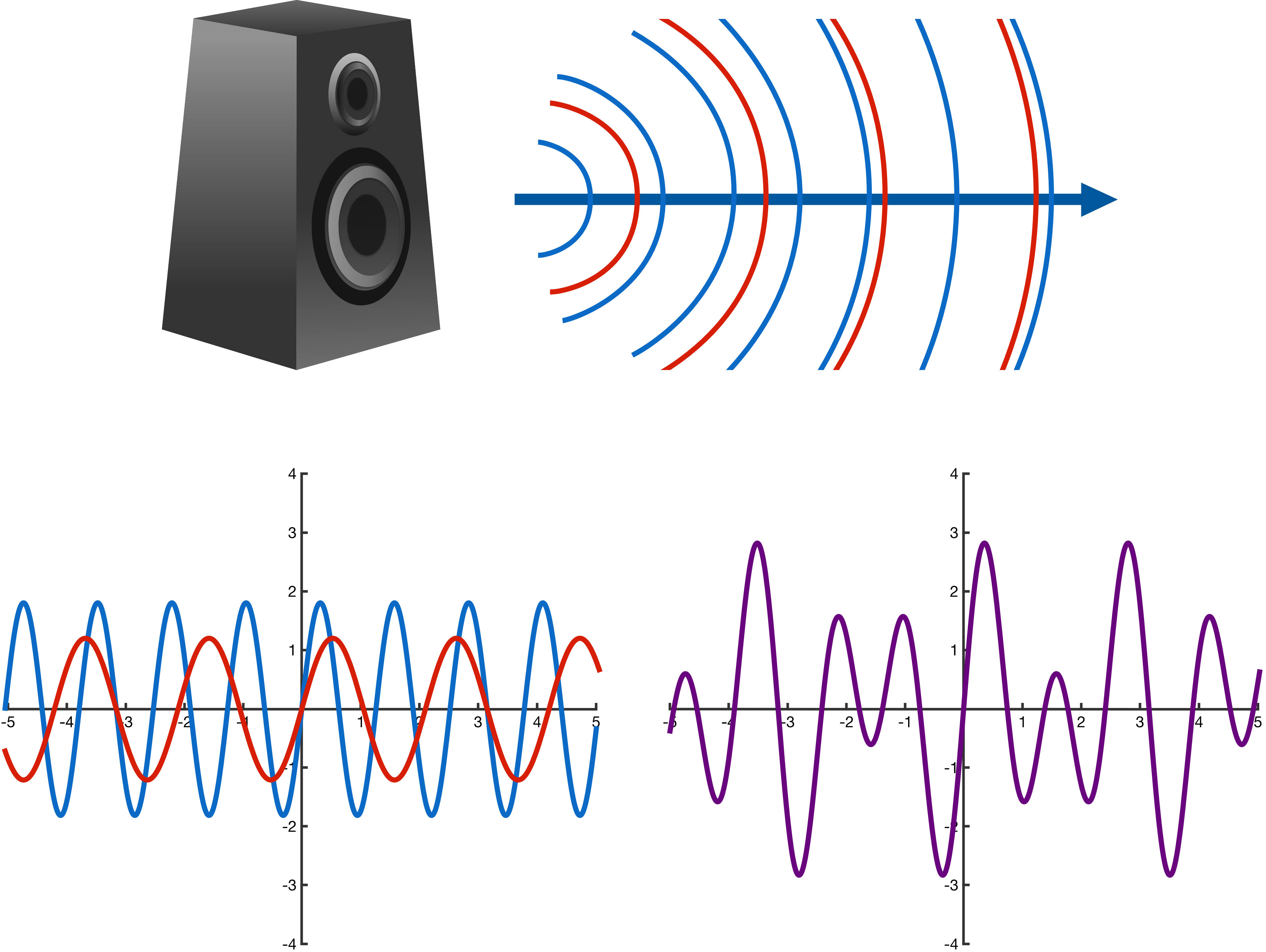Later in this course, you’ll learn a lot more about sine (and cosine) waves and about how to work with them algebraically as well as intuitively. If you like, you can play with a pair of sine waves below and see what they look like when combined (the meaning of the different variables will be explored during the course).

×